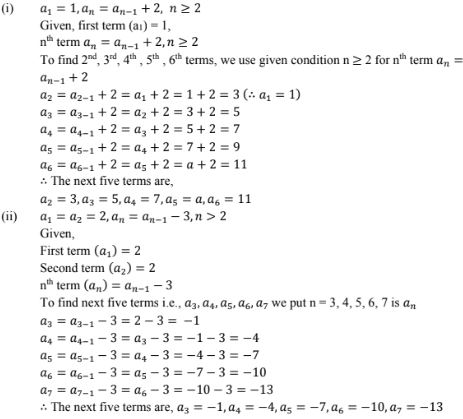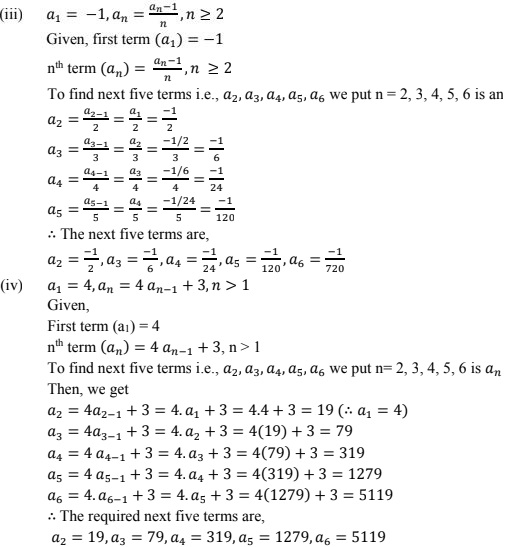# RD Sharma Solutions Chapter 9 Arithmetic Progression Exercise 9.1 Class 10 Maths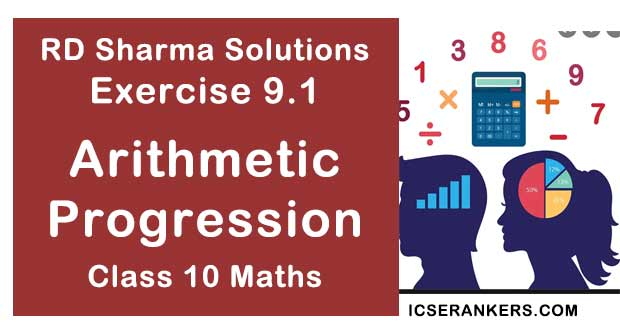Chapter Name RD Sharma Chapter 9 Arithmetic Progression Book Name RD Sharma Mathematics for Class 10 Other Exercises Exercise 9.2Exercise 9.3Exercise 9.4Exercise 9.5MCQ Related Study NCERT Solutions for Class 10 Maths

### Exercise 9.1 Solutions

1. Write the first terms of each of the following sequences whose n th term are
(i) an = 3n + 2
(ii) an = (n - 2)/3
(iii) an = 3n .
(iv) an = (3n - 2)/5
(v) an = (-1)n 2n
(vi) an = n(n - 2)/2
(vii) an = n2 - n + 1
(viii) an = n2 - n + 1
(ix) an = (2n - 3)/6

Solution

We have to write first five terms of given sequences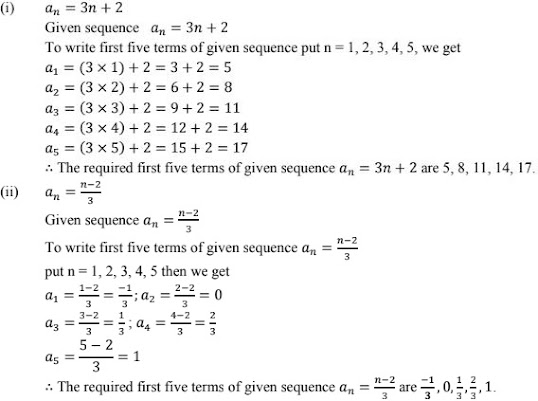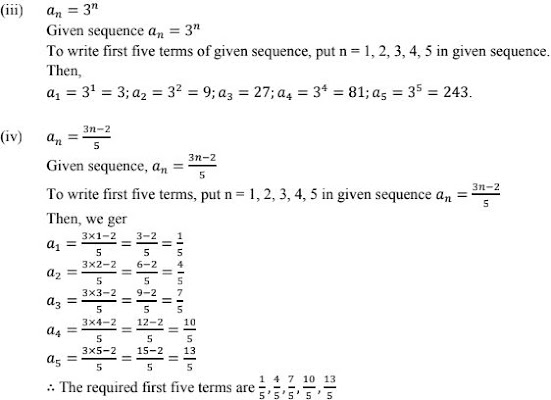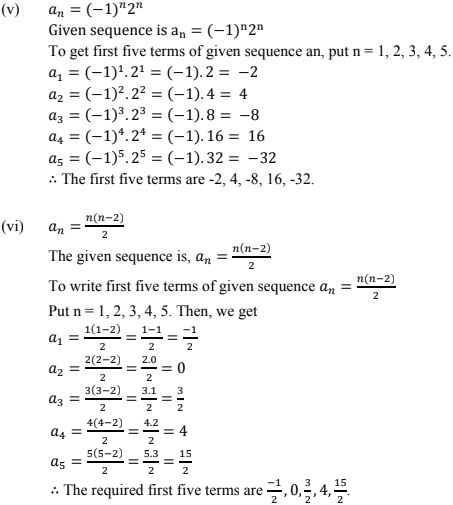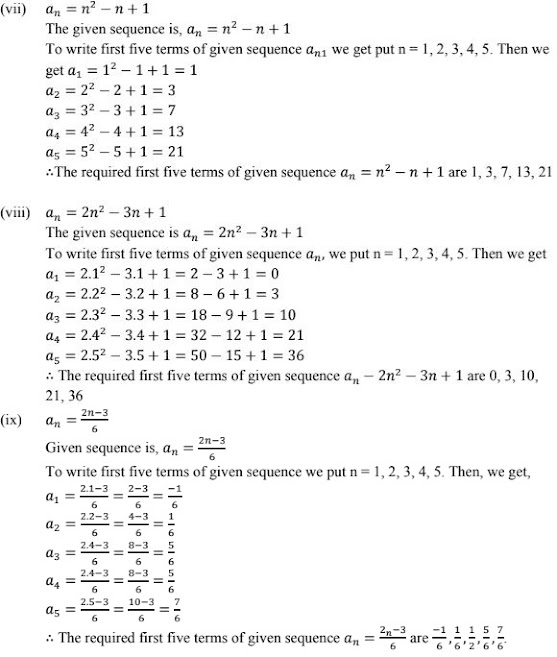2. Find the indicated terms in each of the following sequences whose nth terms are:
(i)  an = 5n - 4;  a12 and  a15
(ii)  an = (3n - 2)/(4n + 5);  a7 and  a8
(iii)  an = n(n-1)(n-2);  a5 and  a8
(iv)  an = (n - 1)(2 - n)(3 + n);  a11  a21  a3
(v)  an = (-1)n n;  a3 , a5 , a8
Solution
We have to find the required term of a sequence when nth term of that sequence is given.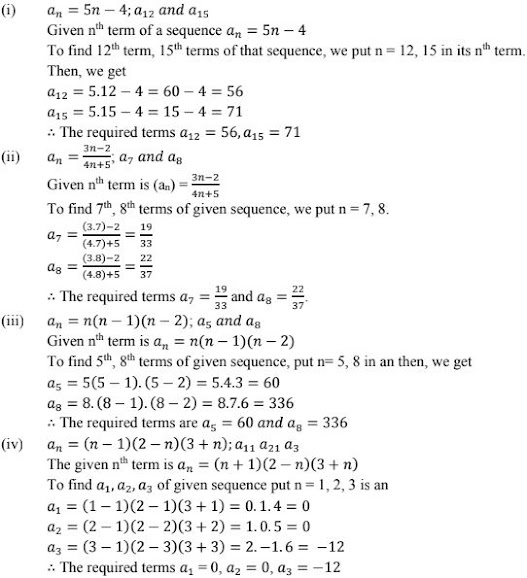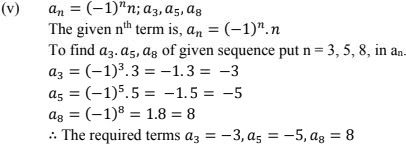3. Find the next five terms of each of the following sequences given by:
(i) a1 = 1,  an = an - 1 + 2, n ≥ 2
(ii) a1 = a2 = 2 , an = an - 1 - 3, n > 2
(iii) a1 = -1,  an = (an - 1 )/n , n ≥ 2
(iv) a1 = 4,  an = 4 an - 1 + 3, n > 1
Solution
We have to find next five terms of following sequences.